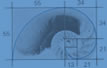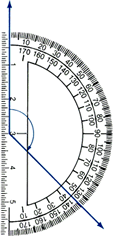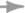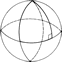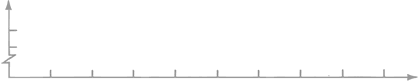Here's what we are learning in math this year!

Common Core for 5th Grade Math

Recommended 5th Grade Math Skills

Math
Mrs. K's Class
Math homework policy: Math will be due the following school day so we can go over the answers in class and discuss any questions or areas of difficulty. This is homework, it does not need to be perfect but it does need to be quality work. I will do my best not to send too much homework at one time so if your child finishes early please have them practice any quick facts like multiplication, division, or anything they need a bit more practice with. If you feel like your child needs more practice items please do not hestiate to ask!

Chapter 1: 9/7-9/15: Read and write multi-digit whole numbers; Place value
relationships and comparison; Round whole numbers

1.1 Numbers through one million
1.2 Place value relationships
1.3 Compare whole numbers
1.4 Round whole numbers
1.5 Construct arguments

Vocabulary:
Place value is the position of a digit in a number that tells the value of the digit.
A conjecture is a statement that is believed to be true but has not been proven.
In a number, a period is a group of three digits, separated by commas, starting from the right.
Rounding is a process that determines which multiple of 10, 100, 1,000, and so on, a number is closest to.
A number written as the sum of the values of its digits is written in expanded form. (300,000 + 20,000 + 100 + 30 + 7)
In a number sentence, a symbol that points toward a lesser number or expression is called a less than symbol. <
In a number sentence, a symbol that points toward a greater number or expression is called a greater than symbol. >
OTHER:
There will also be pop quizzes. These will be timed for either a minute or two depending upon how many questions there are. This is meant to encourage the students to practice their automaticity of facts.

Great games to play at home to help are simple cards games. A game where you each flip over one card and add the two cards together as quickly as possible and whom ever gets it correct the quickest wins the cards. You can do this with subtraction and multiplication as well.